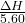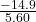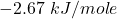Question

If 5.60 mol of a substance releases 14.9 kJ when it decomposes, what is ΔH for the process in terms of kJ/mol of reactant?

1.The correct approach is “-2.67 kJ/mole“. A further solution is described below.

Explanation:

The given values are:

No. of moles,

= 5.60 mol

Substance releases,

ΔH = 14.9 kJ

Now,

The ΔH in terms of kJ/mol will be:

=On putting the given values of ΔH, we get

==